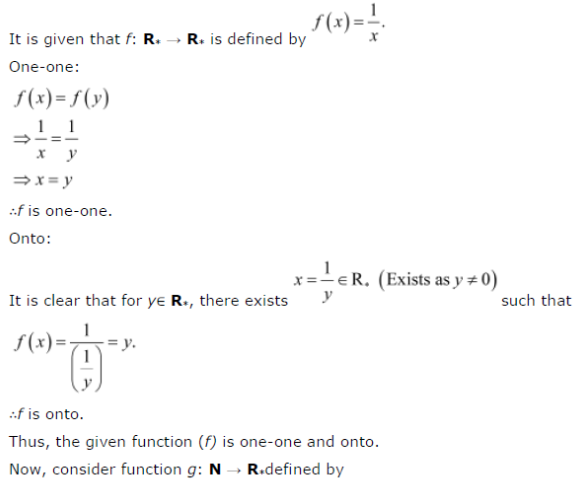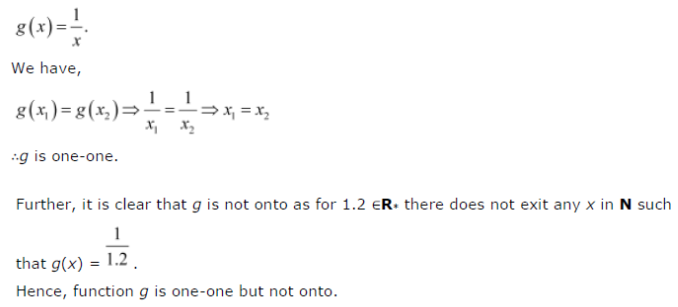# Show that the function f : R* → R* defined by f(x) = 1/x is one-one and onto,where R* is the set of all non-zero real numbers

Show that the function f : R* → R* defined by f(x) = 1/x is one-one and onto,where R* is the set of all non-zero real numbers. Is the result true, if the domain R* is replaced by N with co-domain being same as R* ?